Aptitude Tests 4 Me

Non Verbal Logic

Detailed solution

21.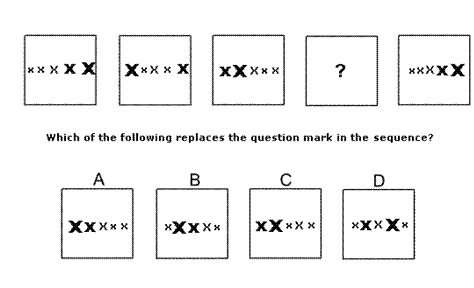Solution: D Explanation: In this question there are 5 different sized letter X's. There are two rules to follow that are applied simultaneously. The first rule is that the X's in the second and third positions (counting from the left) change positions. The second rule is that the fifth X moves to the first position and the others all move along one position towards the right. These two rules are applied simultaneously. Following these rules, the missing diagram of the sequence is D.

22.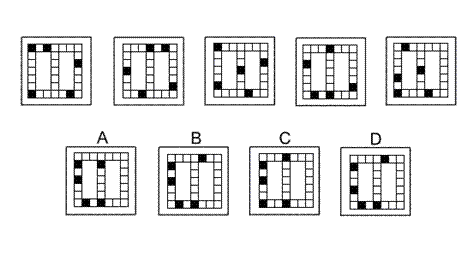Solution: B Explanation: In this question the black squares move three places clockwise around the outside of the grid. The only exception is when a black square lands on the centre square at the top of the grid. In this case, in the next diagram of the sequence, it moves down the centre line of the grid by three places. When it reaches the centre square of the bottom of the grid, it then continues clockwise around the outside of the grid. The correct answer, therefore, is B.

23.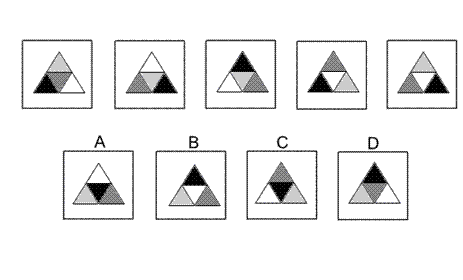Solution: A Explanation: In this question there are two rules to follow that are applied alternately. The first rule is that the colours change - black changes to dark grey, dark grey changes to light grey, light grey changes to white, and white changes to black. The second rule is that the triangular grid rotates by 120° anticlockwise. To obtain the next diagram in the sequence, the first rule should be applied, and the correct answer is A.

24.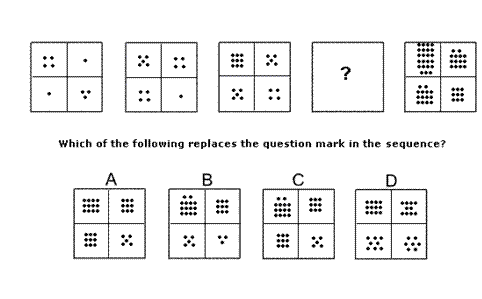Solution: C Explanation: In this question there are two rules to follow that are applied simultaneously. The first rule is that the dots in the first row move down to the second row. The second rule is that for each column, the dots are added and the totals placed in the corresponding squares of the top row. When these two rules are applied simultaneously, the correct diagram to replace the ? is C.

25.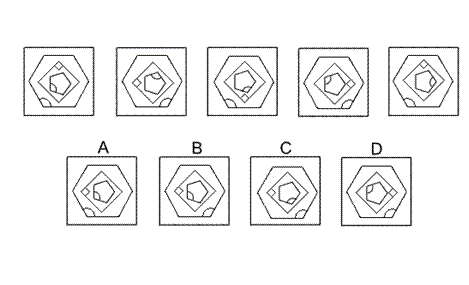Solution: B Explanation: In this question there is a regular pentagon inside a square inside a regular hexagon. Each shape follows its own rule. The regular pentagon rotates by 144° clockwise each time. The square rotates by 90° anticlockwise each time. The regular hexagon is reflected in its vertical axis of symmetry each time. When these rules are applied simultaneously, the next diagram in the sequence is B.Passage ReadingVerbal LogicNon Verbal LogicNumerical LogicData InterpretationReasoningAnalytical AbilityBasic NumeracyAbout UsContactPrivacy PolicyMajor TestsFAQ